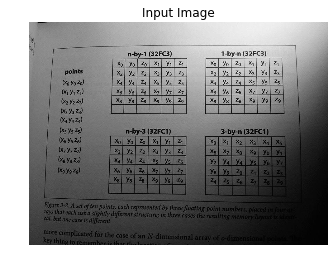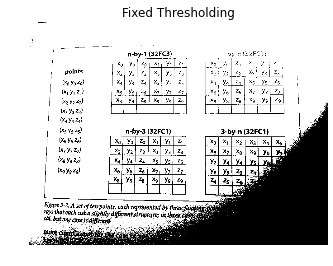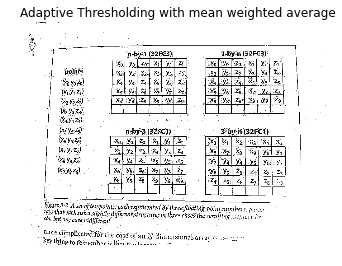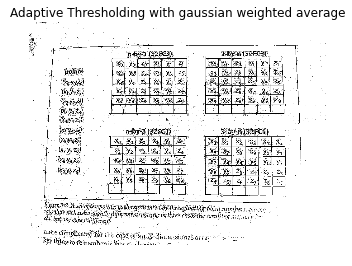# Adaptive Thresholding¶

### Import the required modules¶

Import the required modules.

In :
%pylab inline
import cv2

Populating the interactive namespace from numpy and matplotlib


### Read the input image¶

First of all read the image, then convert the image to grayscale and finally display the image.

In :
im = imread("/home/bikz05/Desktop/image.jpg")
im_gray = cv2.cvtColor(im, cv2.COLOR_RGB2GRAY)
axis("off")
title("Input Image")
imshow(im_gray, cmap = 'gray')
show()### Fixed thresholding¶

We will first perform fixed thresholding on the input image. Fixed Thresholding is done using the function cv2.threshold. The signature of cv2.threshold is -

cv2.threshold(src, thresh, maxval, type[, dst]) -> retval, dst


Here,

1. src is the input image.
2. thresh is the threshold value.
3. maxval is the maximum value that can be assigned to the output.
4. type is the type of thresholding.
5. dst is the destination image

The threshold value set is 50 and the threshold type is cv2.THRESH_BINARY i.e. any value that is greater than 50 is set to 255 and any value less than 55 is set to 0.

In :
retval, im_at_fixed = cv2.threshold(im_gray, 50, 255, cv2.THRESH_BINARY)
axis("off")
title("Fixed Thresholding")
imshow(im_at_fixed, cmap = 'gray')
show()### Adaptive Thresholding with mean weighted average.¶

Adaptive Thresholding with mean weighted average is done using the function cv2.adaptiveThreshold. The signature of the function is -

cv2.adaptiveThreshold(src, maxValue, adaptiveMethod, thresholdType, blockSize, C[, dst]) -> dst


Here,

1. src is the input image.
2. maxval is the maximum value that can be assigned to the output.
3. adaptiveMethod is set to cv2.ADAPTIVE_THRESH_MEAN_C for mean weighted average and to cv2.ADAPTIVE_THRESH_GAUSSIAN_C for gaussian weighted average.
4. thresholdType - the type of thresholding
5. blockSize - value of b
6. C - the constant that is subtracted from the threshold value calculated for each pixel
7. dst is the destination image

For the example, the value of b is 5 and the constant value is set to 10.

In :
im_at_mean = cv2.adaptiveThreshold(im_gray, 255, cv2.ADAPTIVE_THRESH_MEAN_C, cv2.THRESH_BINARY, 5, 10)
axis("off")
title("Adaptive Thresholding with mean weighted average")
imshow(im_at_mean, cmap = 'gray')
show()### Adaptive Thresholding with gaussian weighted average¶

Adaptive Thresholding with gaussian weighted average is done using the function cv2.adaptiveThreshold. The signature of the function is -

cv2.adaptiveThreshold(src, maxValue, adaptiveMethod, thresholdType, blockSize, C[, dst]) -> dst


Here,

1. src is the input image.
2. maxval is the maximum value that can be assigned to the output.
3. adaptiveMethod is set to cv2.ADAPTIVE_THRESH_MEAN_C for mean weighted average and to cv2.ADAPTIVE_THRESH_GAUSSIAN_C for gaussian weighted average.
4. thresholdType - the type of thresholding
5. blockSize - value of b
6. C - the constant that is subtracted from the threshold value calculated for each pixel
7. dst is the destination image

For the example, the value of b is 5 and the constant value is set to 7.

In :
im_at_gauss = cv2.adaptiveThreshold(im_gray, 255, cv2.ADAPTIVE_THRESH_GAUSSIAN_C, cv2.THRESH_BINARY, 5, 7)
axis("off")
title("Adaptive Thresholding with gaussian weighted average")
imshow(im_at_gauss, cmap = 'gray')
show()That's it! Clearly results are better with adaptive thresholding as compared to fixed thresholding.